# Finding patterns in shapes Online Quiz

Following quiz provides Multiple Choice Questions (MCQs) related to Finding patterns in shapes. You will have to read all the given answers and click over the correct answer. If you are not sure about the answer then you can check the answer using Show Answer button. You can use Next Quiz button to check new set of questions in the quiz.Q 1 - The sequence of figures shows a pattern. One flower has 8 petals. If the pattern repeats, how many petals will 7 flowers have?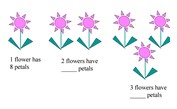### Explanation

Step 1:

In given pattern each flower has 8 petals.

Step 2:

So, 7 flowers should have 7 × 8 = 56 petals.

Q 2 - The sequence of figures shows a pattern. If the pattern repeats, how many dots will figure 4 have?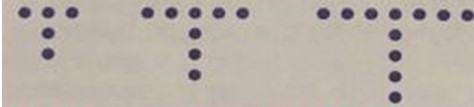### Explanation

Step 1:

In given pattern horizontal dots in numbers are 3, 5, 7 and vertical dots in numbers are 2, 3, 4

Step 2:

So, figure 4 will have 9 horizontal dots and 5 vertical dots. A total of 9 + 5 = 14 dots.

Q 3 - The sequence of 3 figures shows a pattern. If the pattern repeats, how many marbles will there be in figure 5?### Explanation

Step 1:

In given pattern horizontal marbles are always 3 in number and vertical marbles in numbers are 1, 2, 3

Step 2:

So, figure 5 will have 3 horizontal marbles and 5 vertical marbles. A total of 3 + 5 = 8 marbles.

Q 4 - The sequence of 3 figures shows a pattern. If the pattern repeats, how many triangles will the figure 5 have?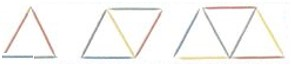### Explanation

Step 1:

The number of triangles in the three figures are 1, 2, and 3. This is the pattern.

Step 2:

As per the pattern, the figure 5 should have 5 triangles.

Q 5 - The sequence of figures shows a pattern. If the pattern repeats, how many small circles will figure 4 have?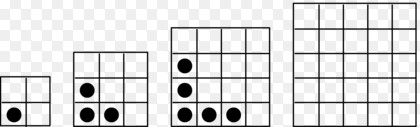### Explanation

Step 1:

The number of small circles in the three figures are 1, 3, and 5. These numbers are consecutive odd numbers.

Step 2:

As per the pattern, the figure 4 should have 7 small circles.

Q 6 - The sequence of figures shows a pattern. If the pattern repeats, how many small squares will figure 4 have?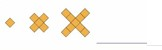### Explanation

Step 1:

The number of small squares in the three figures are 1, 5, and 9. This is an arithmetic sequence whose common difference is 4.

Step 2:

As per the pattern, the figure 4 should have 9 + 4 or 13 small squares.

Q 7 - The sequence of figures shows a pattern. If the pattern repeats, how many small squares will figure 5 have?### Explanation

Step 1:

The number of small squares in the three figures are 2, 4, and 6. This is an arithmetic sequence whose common difference is 2.

Step 2:

As per the pattern, the figure 5 should have 6 + 2 + 2 or 10 small squares.

Q 8 - The sequence of figures shows a pattern. If the pattern repeats, how many small boxes will figure 3 have?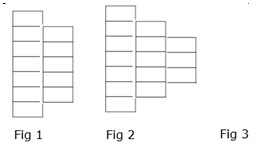### Explanation

Step 1:

The number of small boxes in the two figures are 7 + 5, and 7 + 5 + 3. This is an arithmetic sequence having decreasing odd numbers.

Step 2:

As per the pattern, the figure 3 should have 7 + 5 + 3 + 1 or 16 small boxes.

Q 9 - The sequence of figures shows a pattern. If the pattern repeats, how many smoke signs will Stage 5 have?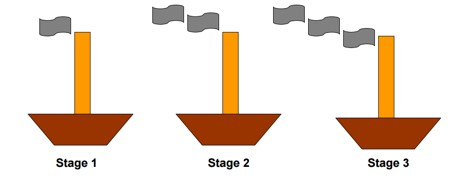### Explanation

Step 1:

The number of smoke signs in the three stages are 1, 2, and 3. This is an arithmetic sequence whose common difference is 1.

Step 2:

As per the pattern, the stage 5 should have 3 + 1 + 1 or 5 smoke signs.

Q 10 - The sequence of figures shows a pattern. If the pattern repeats, how many bubbles will Stage 6 have?